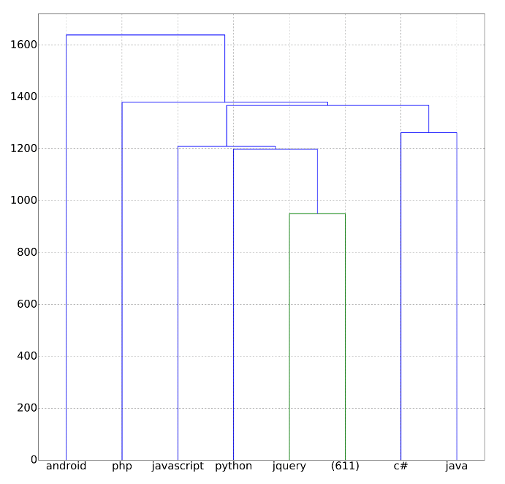Quick Reminder: Clustering

# Quick Reminder: Clustering

Last updated:

## Traditional (non-hierachical) Clusters, such as K-Means:

Need to be given the number N of clusters and the initial cluster positions (centroids).

## Hierarchical clusters

No need to inform number of clusters and positions, but you need to inform the linkage type.

Can be agglomerative (bottom-up) or divisive (top-down).

It's the measure of dissimilarity (distance) between clusters.

• single linkage: distance between two groups is the smallest distance between two points in these groups.

• elements at opposite ends of a cluster may be much farther from each other than to elements of other clusters.
• complete linkage: distance between two groups is the largest distance between two points in these groups.

• Favours compact clusters with small diameters over long, straggly clusters.
• Sensitive to outliers.
• average linkage: distance between two groups is the average distance between two points in these groups.

• ward linkage: distance between two groups is the difference between the sum of the squared distances of all points within each group.

• Similar to K-means

## Dendrograms

It's a way of representing hierarchical clusters.

Y-axis indicates dissimilarity.

E.g.,in the following picture:

• the dissimilarity between android and all other concepts is a little over 1600.

• the dissimilarity between php and javascript is around 1400.

• the dissimilarity between c# and java is a little over 1200.Sample dendrogram (single linkage) of programming concepts.
Created using scipy.cluster.hierarchy.dendrogram